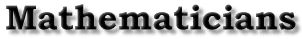| The Abstractor | Elementary Notions | A Fourth Dimension | A Division Algebra | Closure | An Example of Hamilton's Work | Bibliography | Back to the front page A Division Algebra Joseph Johnson Hamilton had discovered what modern mathematicians term a skew field. That is, the system satisfies the following axioms applied to the direct sum of four real additive groups, under Hamilton's definitions of equality, addition, and multiplication. Let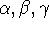be any quaternions with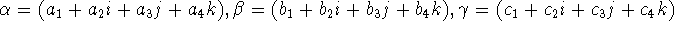(1) Closure of (a) addition and (b) multiplication. Both the addition and multiplication of two quaternions yields another quaternion. (2) Commutativity of (a) addition and falsity of (b) multiplication commutativity. It is easy to see that. Of course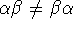in general. (3) Associativity of (a) addition and (b) multiplication. Clearly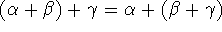, by properties of real numbers. The proof of multiplication associativity has been described as ``torture" by at least one mathematician. (4) (a) Addititive and (b) multiplicative identities. The addititve identity is simply 0.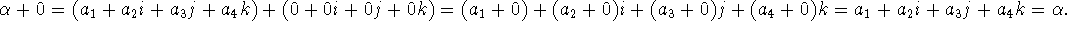The mulitiplicative identity is 1=(1+0i+0j+0k). (5) (a) Additive and (b) multiplicative inverses. The additive inverse of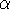is simply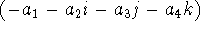. Finding the multiplicative inverse ofis more detailed. The use of the aforementioned modulus function,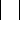, is crucial.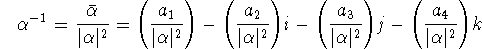where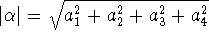. This can be derived as a consequence of conjugacy in Quaternions (see Elements Paragraph 187). Every quaternionhas a conjugate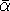such that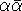is a real number. This allows us to set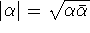. The explicit conjugate of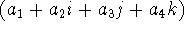is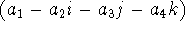(which is analogous to conjugacy of complex numbers). (6) Distributive laws. It seems almost amazing that the Quaternions are in fact distributive. That isIf this axiom did not hold, the Quaternions would be utterly uninteresting. It would represent two separate group structures (after deleting 0 in the multiplicative case) - hardly impressive to most mathematicians. | The Abstractor | Elementary Notions | A Fourth Dimension | A Division Algebra | Closure | An Example of Hamilton's Work | Bibliography | Back to the front page